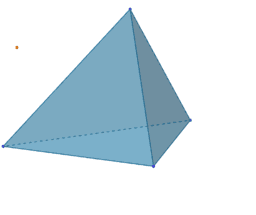# ₈Polyhedra with extreme distribution of equivalent vertices

For a given number of n particles on a sphere, we consider only polyhedrons whose vertices, firstly, are extremely distributed and, secondly, equivalent to each other. By extreme distributions, we mean the distribution of points on the sphere that correspond to the local extrema (maxima) of Distance Sum. The sum of distances is measured by summing all the segments connecting each possible combination of 2 points. The "measure" of this distribution is the average distance between particles on the unit sphere(pn). The method of Lagrange multipliers is used to find the extreme distributions of particles on a sphere. *From Book: Extended definitions of point location estimates https://www.geogebra.org/m/hhmfbvde From: List of My Public Books on GeoGebra Topics: Constructing polyhedra -https://www.geogebra.org/m/eabstecp
•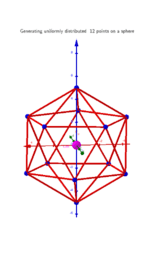### Polyhedra whose vertices are equivalent and have an extreme distribution on the same sphere.

•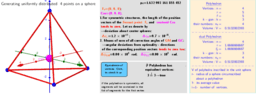### Tetrahedron,n=4. Polyhedra with extreme distribution of equivalent vertices.

•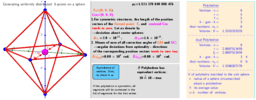### Octahedron, n=6. Polyhedra with extreme distribution of equivalent vertices.

•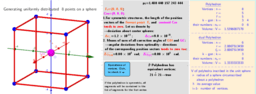### Cube, n=8. Polyhedra with extreme distribution of equivalent vertices.

•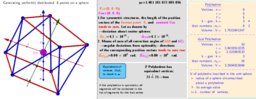### Square antiprism, n=8. Polyhedra with extreme distribution of equivalent vertices.

•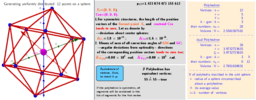### Icosahedron, n=12. Polyhedra with extreme distribution of equivalent vertices.

•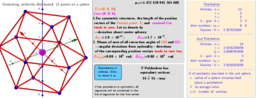### Cuboctahedron, n=12. Polyhedra with extreme distribution of equivalent vertices.

•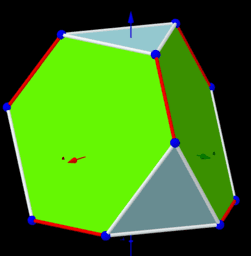### Truncated Tetrahedron, n=12. Polyhedra with extreme distribution of equivalent vertices.

•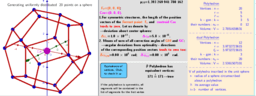### Dodecahedron, n=20. Polyhedra with extreme distribution of equivalent vertices.

•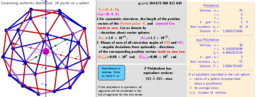### Biscribed Snub Cube(laevo), n=24. Polyhedra with extreme distribution of equivalent vertices.

•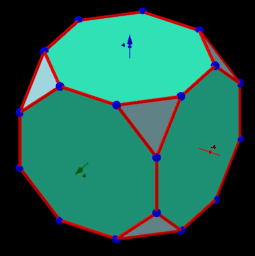### Truncated Cube, n=24. Polyhedra with extreme distribution of equivalent vertices.

•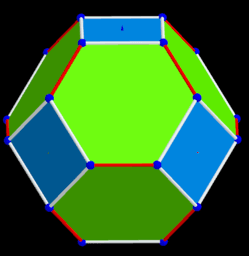### Biscribed Truncated Octahedron, n=24. Polyhedra with extreme distribution of equivalent vertices.

•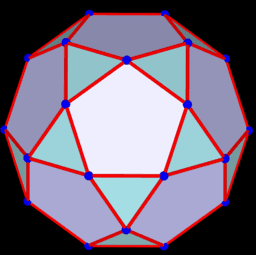### Icosidodecahedron, n=30. Polyhedra with extreme distribution of equivalent vertices.

•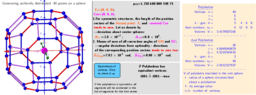### Biscribed Truncated Cuboctahedron, n=48. Polyhedra with extreme distribution of equivalent vertices.

•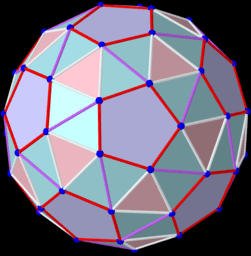### Biscribed Snub Dodecahedron, n=60. Polyhedra with extreme distribution of equivalent vertices.

•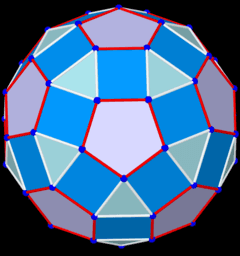### Rhombicosidodecahedron, n=60. Polyhedra with extreme distribution of equivalent vertices.

•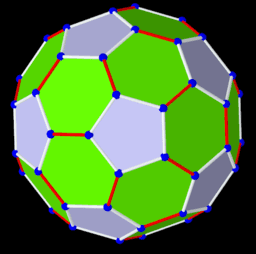### Biscribed Truncated Icosahedron, n=60. Polyhedra with extreme distribution of equivalent vertices.

•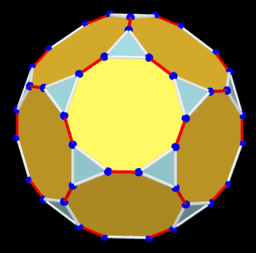### Truncated Dodecahedron, n=60. Polyhedra with extreme distribution of equivalent vertices.

•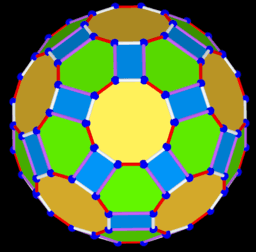### Biscribed Truncated Icosidodecahedron, n=120. Polyhedra with extreme distribution of equivalent vertices.

•### ddddd

•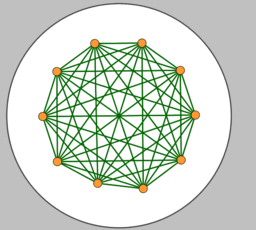### Equilibrios dinámicos

•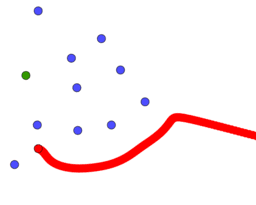### Robot entre enemigos

•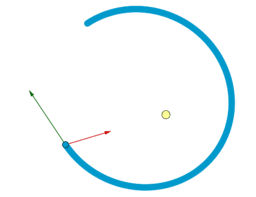### Órbitas elípticas

•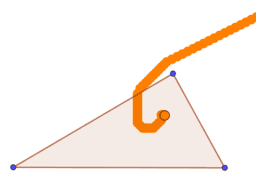### Robot punto Fermat

•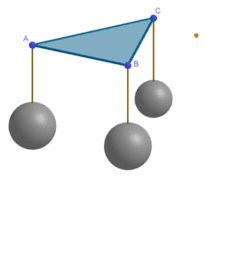•